# 1.1. Draw¶

There are two usual methods for light waves polarization representation: polarization ellipse and Poincaré sphere.

:

%load_ext autoreload
import numpy as np
from py_pol.jones_vector import Jones_vector, degrees
from py_pol.stokes import Stokes


## 1.1.1. Polarization ellipse¶

### 1.1.1.1. Pure states¶

The trajectory of the electric field vector of pure (totally polarized) light waves is an ellipse. This ellipse is often used to represent polarization states, as it allows identifying easily some of its properties. py_pol has a method in Jones_vector and Stokes classes to plot the polarization ellipses: draw_ellipse.

:

J = Jones_vector('Circular light')
J.circular_light(intensity=2, kind='r')
print(J)
axis, fig = J.draw_ellipse(draw_arrow=True, figsize=(5,5))

Circular light =
[+1.000+0.000j]
[+0.000+1.000j]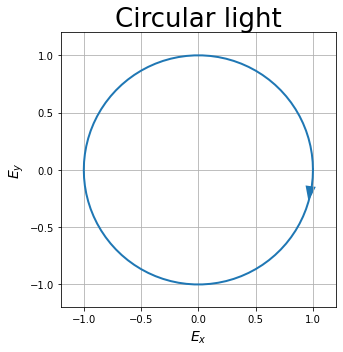Same for Stokes objects.

:

S = Stokes('Circular light')
S.circular_light(intensity=2, kind='r')
print(S)
axis, fig = S.draw_ellipse(draw_arrow=True, figsize=(5,5))

Circular light =
[+2.000]
[+0.000]
[+0.000]
[+2.000]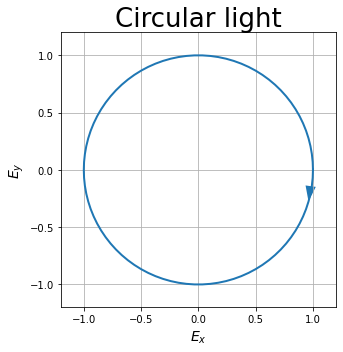### 1.1.1.2. Plot multiple ellipses in a single plot¶

py_pol objects may have more than one element stored inside. The most basic method of representation consists on plotting all the ellipses in the same figure:

:

# It is the same for Stokes class
S = Stokes('Elliptical light')
angles = np.linspace(0, 180*degrees, 7)
S.general_azimuth_ellipticity(amplitude=2, azimuth=angles, ellipticity=15*degrees)
S.draw_ellipse(draw_arrow=True, figsize=(6, 6))

:

(<Figure size 432x432 with 1 Axes>,
[<AxesSubplot:title={'center':'Elliptical light'}, xlabel='$E_x (V/m)$', ylabel='$E_y (V/m)$'>])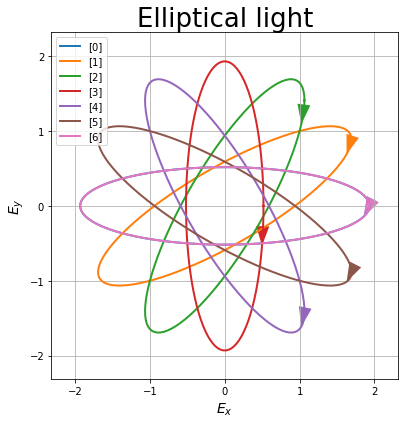### 1.1.1.3. Plot several ellipses in multiple plots¶

It is possible to divide the plots into several ones using the subplots argument. The first option is INDIVIDUAL, which represents each ellipse in its own subplot. These subplots are represented in a square grid (or the closest to a square grid, depending on the number of elements of the object):

:

J = Jones_vector('Linear light')
angles = np.linspace(0, 180*degrees, 5)
J.linear_light(amplitude=2, azimuth=angles)
_ = J.draw_ellipse(draw_arrow=True, subplots='Individual', figsize=(6, 12))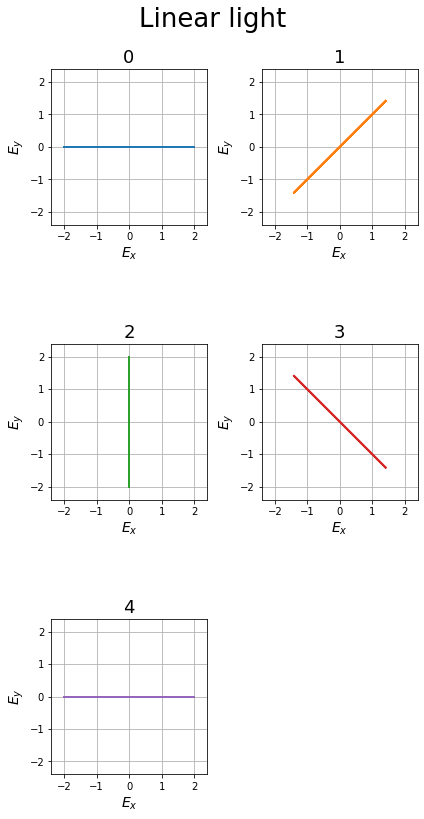The second option is AS_SHAPE, which uses the shape of the object. 1D objects will be plotted as a row array of plots with one ellipse in each subplot. 2D arrays will be plotted as a matrix array with the same shape as the object. Objects of higher dimensionality will be plotted as a matrix array with the shape of the two first dimensions, plotting more than one ellipse per subplot.

:

# 1D object
J = Jones_vector('Linear light')
angles = np.linspace(0, 180*degrees, 5)
J.linear_light(amplitude=2, azimuth=angles)
_ = J.draw_ellipse(draw_arrow=True, subplots='As_shape', figsize=(15, 4))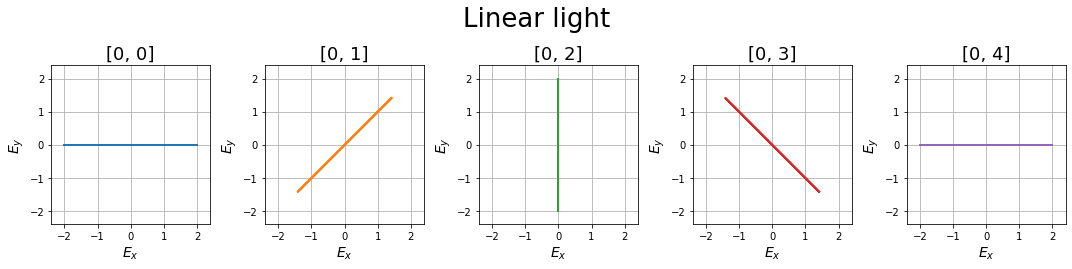:

# 2D object
J = Jones_vector('Light source')
az = np.linspace(0, 90*degrees, 5)
el = np.linspace(0, 45*degrees, 3)
AZ, EL = np.meshgrid(az, el)
J.general_azimuth_ellipticity(amplitude=2, azimuth=AZ, ellipticity=EL)
_ = J.draw_ellipse(draw_arrow=True, subplots='as_shape', figsize=(16, 13))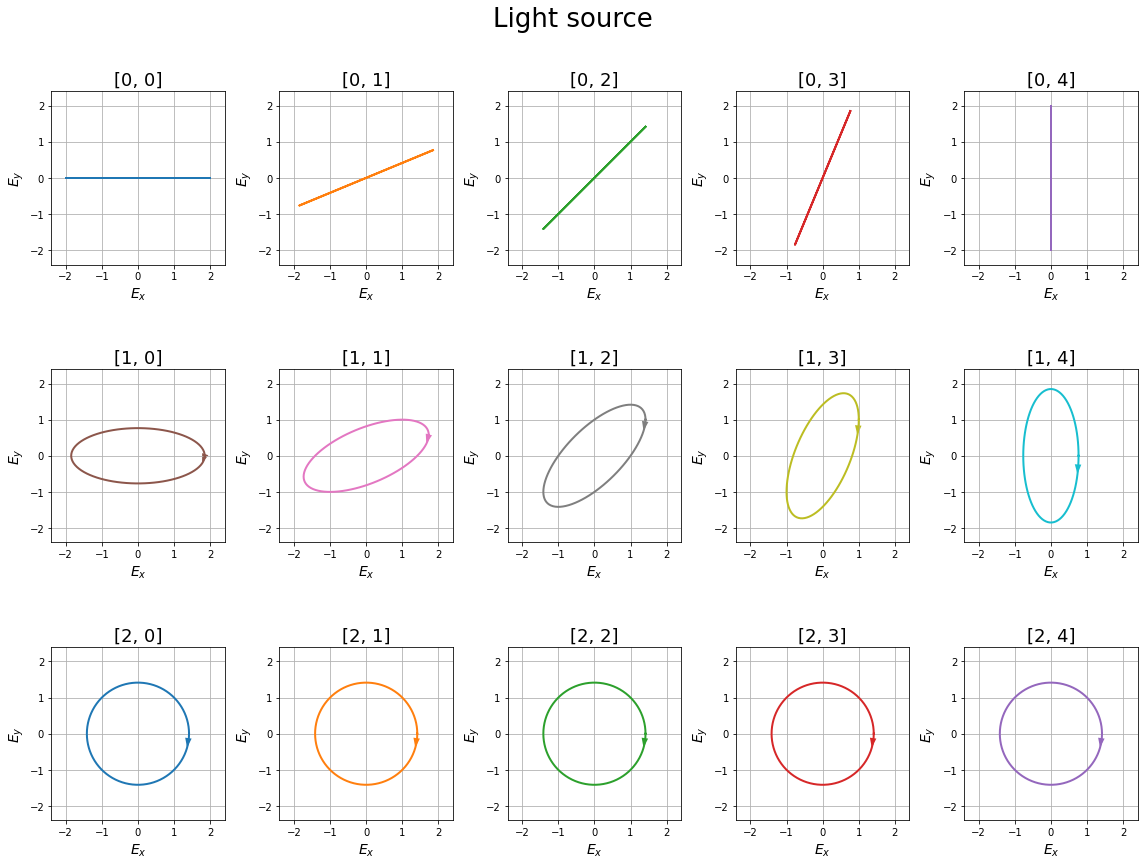:

# Higher dimensionality object
J = Jones_vector('Light source')
angles = np.linspace(0, 90*degrees, 5)
ell = np.linspace(15, 40, 5)*degrees
intensity = np.linspace(1, 3, 3)
Ell, I, Angles = np.meshgrid(ell, intensity, angles)
J.general_azimuth_ellipticity(azimuth=Angles, ellipticity=Ell, intensity=I)
_ = J.draw_ellipse(draw_arrow=True, depol_central=True, subplots='as_shape', figsize=(20, 15))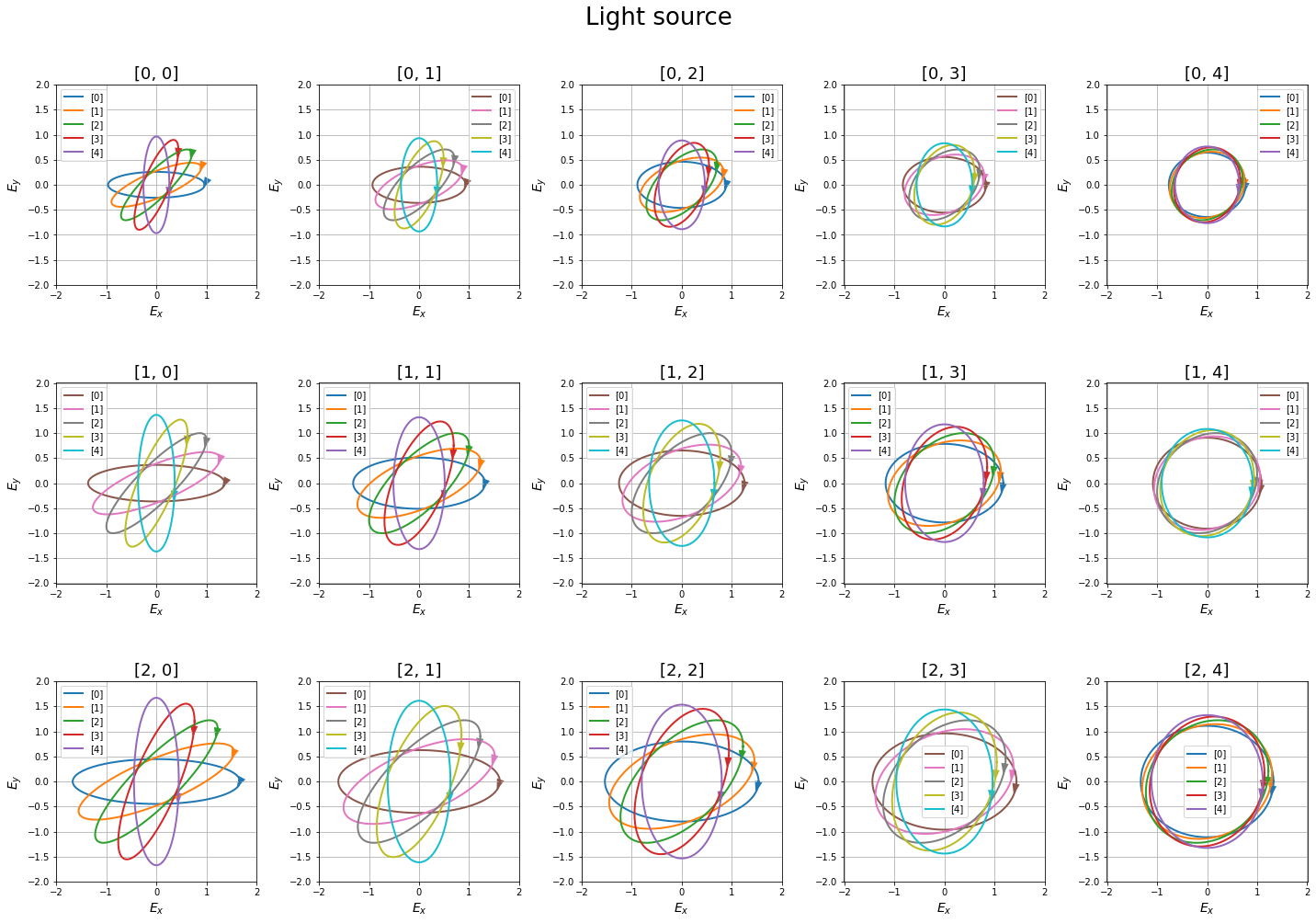Finally, it is possible to give the method a 2-element tuple as the desired shape.

:

J = Jones_vector('Light source')
angles = np.linspace(0, 90*degrees, 10)
ell = np.linspace(15, 40, 6)*degrees
Ell, Angles = np.meshgrid(ell, angles)
J.general_azimuth_ellipticity(azimuth=Angles, ellipticity=Ell, intensity=2)
_ = J.draw_ellipse(draw_arrow=True, depol_central=True, subplots=(3,5), figsize=(20, 15))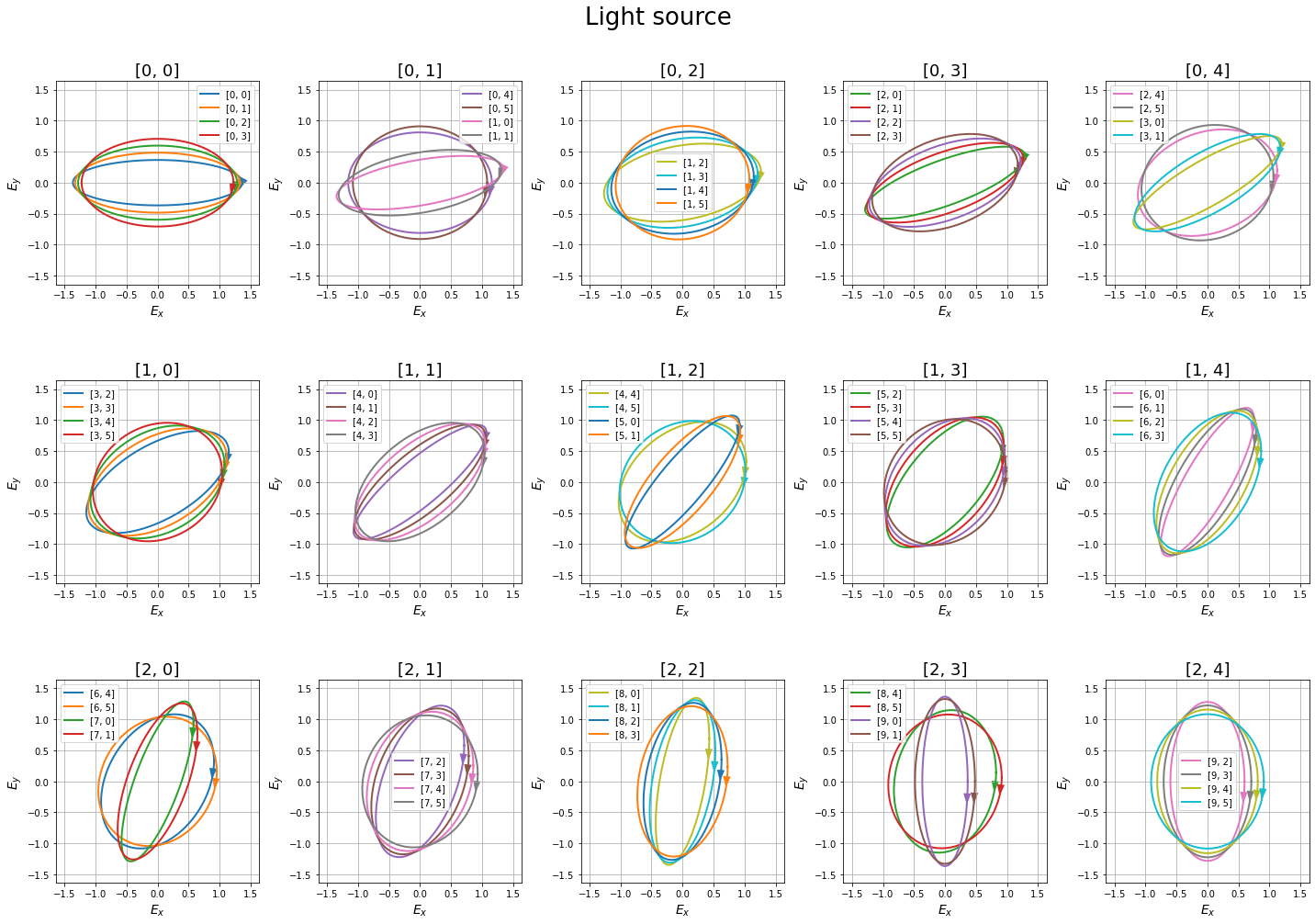### 1.1.1.4. Plot partially polarized states¶

Partially polarized states can be decomposed in a totally polarized state plus a totally depolarized state. The totally unpolarized light electric field vector position will be random, with its module dependent on the unpolarized intensity. Then, the total electric field will be the coherent sum of both fields.

py_pol can plot partially polarized states in three different ways. The first one is plotting at the same time the totally polarized part ellipse and the circle whose radius is the expected electric field amplitude of the unpolarized part. This is done by setting the depol_central argument as True.

:

S = Stokes('Light source')
angles = np.linspace(0, 90*degrees, 5)
ell = np.linspace(15, 40, 5)*degrees
deg = np.linspace(0.2, 0.6, 3)
Ell, Deg, Angles = np.meshgrid(ell, deg, angles)
S.general_azimuth_ellipticity(amplitude=2, azimuth=Angles, ellipticity=Ell, degree_depol=Deg)
# print(J)
_ = S.draw_ellipse(draw_arrow=True, depol_central=True, subplots='as_shape', figsize=(20, 15))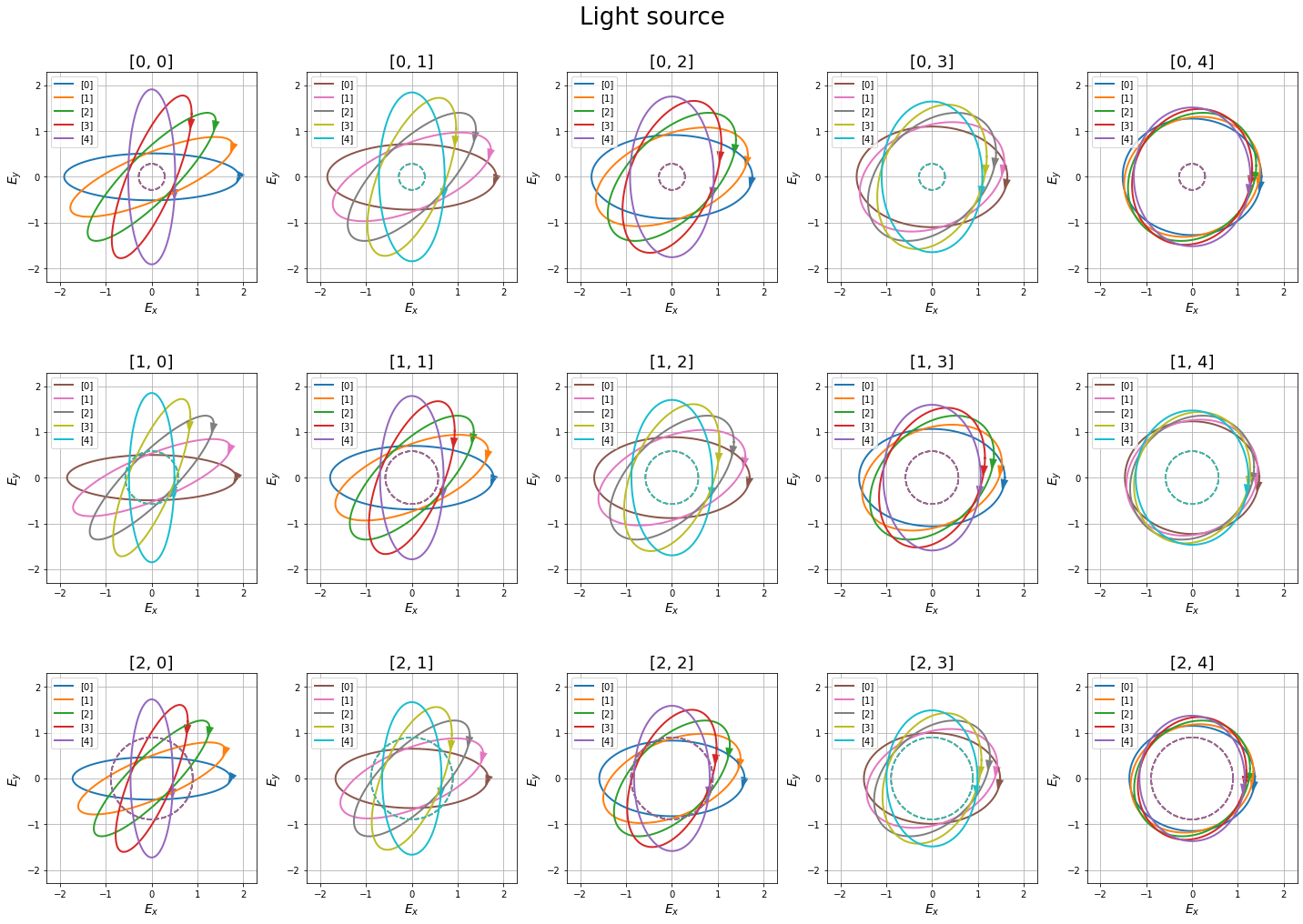The second way is plotting the ellipses which outline the region with a certain probability of finding the total electric field vector. This is done by setting the depol_contour argument as True. The argument contour_levels contains the probability levels which the lines contain. If several ones are used, several lines will be plotted.

:

S = Stokes('Light source')
S.general_azimuth_ellipticity(amplitude=2, azimuth=45*degrees, ellipticity=15*degrees, degree_depol=0.2)
_ = S.draw_ellipse(draw_arrow=True, depol_contour=True, contour_levels=(0.05, 0.2, 0.5,))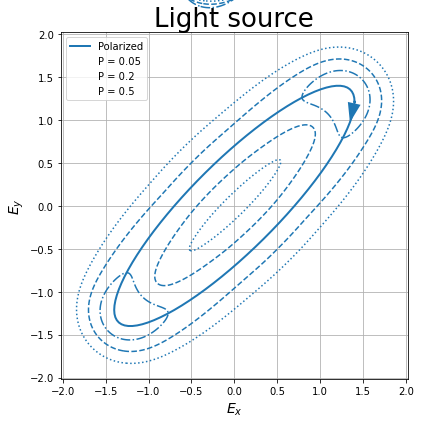The last method is plotting the probability distribution of the electric field. This is done by setting the depol_prob argument to True. The colormap of the probability distribution is varied using the cmap argument.

NOTE: This possibility forces the INDIVIDUAL option of subplots.

:

S = Stokes('Light source')
S.general_azimuth_ellipticity(amplitude=2, azimuth=45*degrees, ellipticity=15*degrees, degree_depol=0.2)
_ = S.draw_ellipse(draw_arrow=True, depol_prob=True, depol_contour=True, contour_levels=(0.05, 0.2, 0.5,))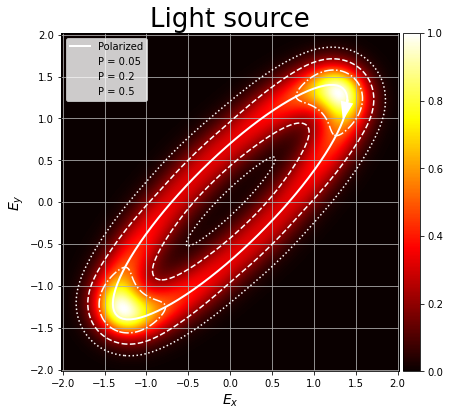## 1.1.2. Poincaré sphere¶

The second representation method is the Poincaré sphere. Each Stokes vector can be represented in a sphere using $$S_1$$, $$S_2$$ and $$S_3$$ as $$x$$, $$y$$ and $$z$$ coordinates. Pure states represent the surface of the wphere, the origin of the sphere for a totally depolarized state (natural light), and the rest of the sphere volume correspond to partially polarized light.

A Stokes object can be plotted in a Poincaré sphere using the draw_poincare method:

:

S = Stokes('Linear light')
S.linear_light(azimuth=0)
print(S)
S.draw_poincare(axis_equal=True)

Linear light =
[+1.000]
[+1.000]
[+0.000]
[+0.000]


:

([<Axes3DSubplot:title={'center':'Linear light'}, xlabel='$S_1$', ylabel='$S_2$'>],
<Figure size 432x432 with 1 Axes>)Again, Stokes objects with more than one vector can be used.

:

S = Stokes('Linear light')
S.linear_light(azimuth=np.linspace(0,180,13)*degrees)
ax, fig = S.draw_poincare()If an object has multiple Stokes vectors, the figure may be divided into subplots using the subplots argument:

:

S = Stokes('Linear light')
S.linear_light(azimuth=np.linspace(0,90,5)*degrees)
ax, fig = S.draw_poincare(figsize=(16,5), subplots='as_shape')py_pol has a powerful option to improve the data representation. By default, Stokes vectors are normalized before plotting them in the Poincaré sphere. This allows comparing the vectors easily, but the intensity information is lost.

But we can set the color_scatter argumento to INTENSITY in order to set the point color to match the intensity.

:

S = Stokes('Linear light')
S.linear_light(azimuth=np.linspace(0,90,5)*degrees, intensity=np.linspace(1,5,5))
ax, fig = S.draw_poincare(figsize=(20,5), subplots='as_shape', color_scatter='Intensity')The images plot points by deffault. However, it is possible to plot the lines between them when more than two points are plotted in the same image by changing the kind argument to LINE or BOTH:

:

S = Stokes('Linear light')
S.linear_light(azimuth=np.linspace(0,90,5)*degrees, intensity=np.linspace(1,5,5))
ax, fig = S.draw_poincare(figsize=(20,5), kind='both', color_scatter='Intensity')## 1.1.3. Other considerations¶

### 1.1.3.1. Save the figure as an image file¶

Finally, it is possible to save the figures if a valid file name is given to the filename argument.

:

S = Stokes('Source 1')
angles = np.linspace(0, 90*degrees, 5)
ell = np.linspace(15, 40, 5)*degrees
deg = np.linspace(0.1, 0.5, 3)
Ell, Deg, Angles = np.meshgrid(ell, deg, angles)
S.general_azimuth_ellipticity(amplitude=2, azimuth=Angles, ellipticity=Ell, degree_depol=Deg)
# print(J)
_ = S.draw_ellipse(draw_arrow=True, depol_central=True, subplots='as_shape', figsize=(20, 15), filename='Subplots figure.jpg')

Image Subplots figure.jpg saved succesfully!